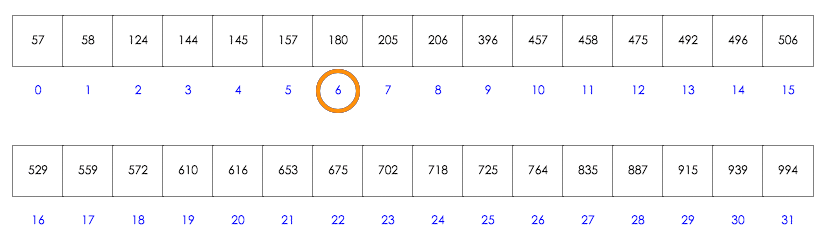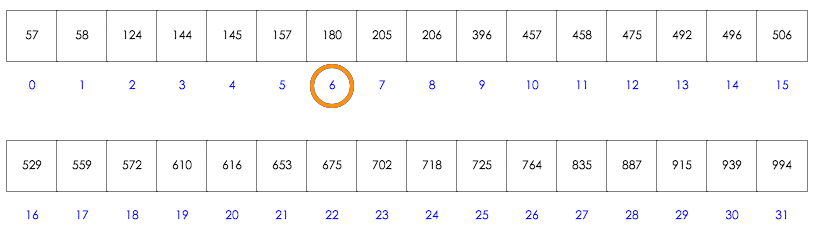# 線性搜尋法

function linear_search(array, target){
for(var i=0; i<array.length; i++){
if(array[i]==target) return i;
}
return -1; //找不到
}# 二分搜尋法

...

1. 決定好左邊界 L，右邊界 R
2. 取 M = (L+R)/2，作為中間的 index
3. 如果 array[M] == 要找的數，return M
4. 如果 array[M]>要找的數，表示 M~R 這一段數字都是不可能的，所以讓 R = M - 1
5. 如果 array[M]<要找的數，表示 L~M 這一段數字都是不可能的，所以讓 L = M + 1
6. 如果 R>=L，持續第 2 步，否則回傳 -1（代表找不到）

R 代表的是：最右邊有可能的值，假如有答案，一定在 <=R 的位置

（藍色是 L，黃色是 R，綠色是 M，要找的數字是 180）function binary_search(array, target) {
var L = 0, R = array.length - 1;
while(L<=R) {
var M = Math.floor((L+R)/2);
if(array[M]==target){
return M;
} else if(array[M]>target) {
R = M - 1;
} else {
L = M + 1;
}
}
return -1;
}


# 不同條件的二分搜尋法

1. 回傳第一個 >=target 的位置
2. 回傳第一個 >target 的位置
3. 回傳最後一個 <=target 的位置
4. 回傳最後一個 <target 的位置

（可參考：lower_bound

function search(array, target){
var L = 0, R = array.length - 1;
while(L<=R) {
var M = Math.floor((L+R)/2);
if(array[M]<target){
L = M;
} else {
R = M - 1;
}
}
return M;
}


array = 3 > 2，所以 R = 2-1 = 1

array = 1 < target，L = M = 0

1. 保證答案一定在閉區間 [L, R] 裡面
2. 當這區間剩下的數很少時，改用線性搜尋

// 傳回最後一個 < target 的數
function lower_bound(array, target) {

// 先看看是否沒答案
// 如果第一個數還是沒有 < target，代表沒答案
if(array>=target) return -1;

// 結束條件是區間內剩兩個以下數字的時候
var L = 0, R = array.length-1;
while((R-L+1)>2) {
var M = Math.floor((L+R)/2);
if(array[M]<target){
L = M;
} else {
R = M - 1;
}
}

// 在答案範圍內用線性搜尋
// 其實到這邊只會剩下兩個元素以內，不一定要用迴圈
for(var i=R; i>=L; i--){
if(array[i]<target){
return i;
}
}
}


# 結論

@huli 野生工程師，相信分享與交流能讓世界變得更美好